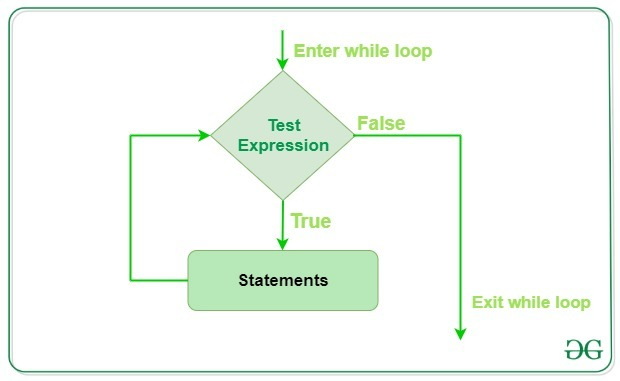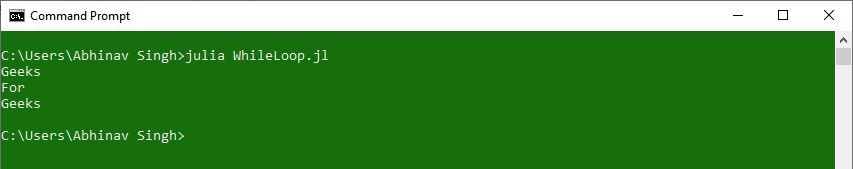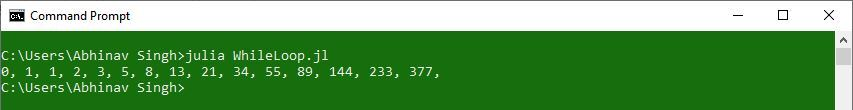Open in App
Not now

# while loop in Julia

• Last Updated : 19 Feb, 2020

In Julia, while loop is used to execute a block of statements repeatedly until a given condition is satisfied. And when the condition becomes false, the line immediately after the loop in the program is executed. If the condition is false when the while loop is executed first time, then the body of the loop will never be executed.Syntax :

```while expression

statement(s)

end
```

Here, ‘while‘ is the keyword to start while loop, ‘expression‘ is the condition to be satisfied, and ‘end‘ is the keyword to end the while loop.

Note: A block of code is the set of statements enclosed between the conditional statement and the ‘end‘ statement.

Example 1:

 `# Julia program to illustrate ``# the use of while loop`` ` `# Declaring Array``Array ``=` `[``"Geeks"``, ``"For"``, ``"Geeks"``]`` ` `# Iterator Variable``i ``=` `1`` ` `# while loop``while` `i <``=` `length(Array)`` ` `    ``# Assigning value to object``    ``Object` `=` `Array[i]``     ` `    ``# Printing object``    ``println(``"\$Object"``)``     ` `    ``# Updating iterator globally``    ``global` `i ``+``=` `1``     ` `# Ending Loop``end`

Output:Example 2:

 `# Julia program to generate ``# the Fibonacci sequence `` ` `# The length of Fibonacci sequence``length ``=` `15`` ` `# The first two values``a ``=` `0``b ``=` `1`` ` `# Iterator Value``itr ``=` `0`` ` `# while loop condition``while` `itr < length`` ` `   ``# Printing fibonacci value``   ``print``(a, ``", "``)``    ` `   ``# Updating value``   ``c ``=` `a ``+` `b``    ` `   ``# Modify values``   ``global` `a ``=` `b``   ``global` `b ``=` `c``    ` `   ``# Updating iterator``   ``global` `itr ``+``=` `1`` ` `# End of while loop``end`My Personal Notes arrow_drop_up